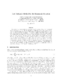## Fast Multipole Method for the Biharmonic EquationThe evaluation of sums (matrix-vector products) of the solutions of the three-dimensional biharmonic equation can be accelerated using the fast multipole method, while memory requirements can also be significantly reduced. We develop a complete translation theory for these equations. It is shown that translations of elementary solutions of the biharmonic equation can be achieved by considering the translation of a pair of elementary solutions of the Laplace equations. Compared to previous methods that required the translation of five Laplace elementary solutions for the biharmonic Green's function, and much larger numbers for higher order multipoles, our method is significantly more efficient. The theory is implemented and numerical tests presented that demonstrate the performance of the method for varying problem sizes and accuracy requirements. In our implementation the FMM\ is faster than direct solution for a matrix size of $550$ for an accuracy of $10^{-3},$ 950 for an accuracy of $10^{-6} and$N=3550$for an accuracy of$10^{-9}\$.